# Plotting functions with Sage!

## Plotting with Sage!

Yesterday we talked briefly about Sage, had you create an account and a project called 'Calculus Center'.  Today we are going to use that project to plot functions.  Let's get started:

• When you get in you should see a listing of your projects.  Click the 'Calculus Center' project link.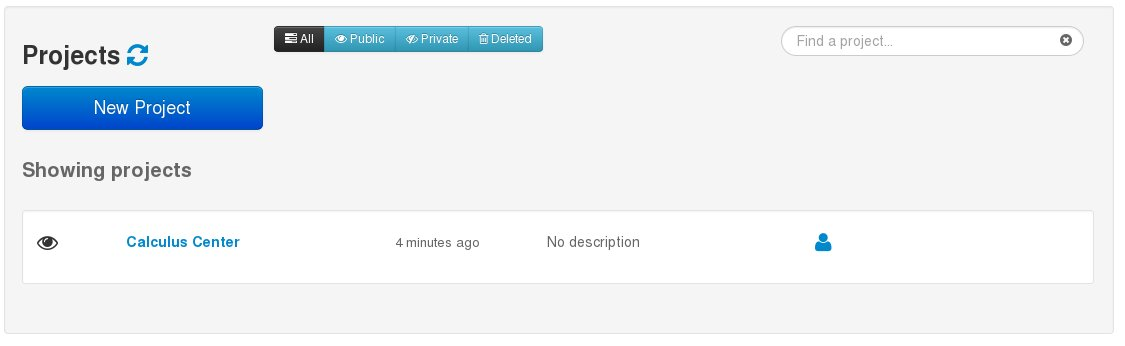• At the top you now want to click the "New" button.• In the text box put the name plot_example and click the "Sage Worksheet" button.  This will create a worksheet called plot_example where we will plot our functions.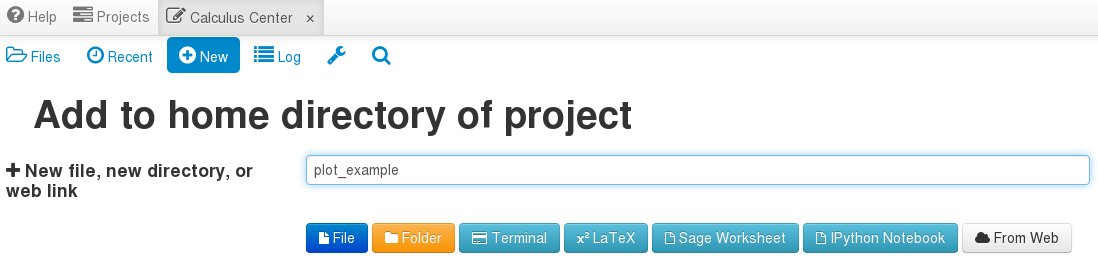• Now you will see a screen that looks like this: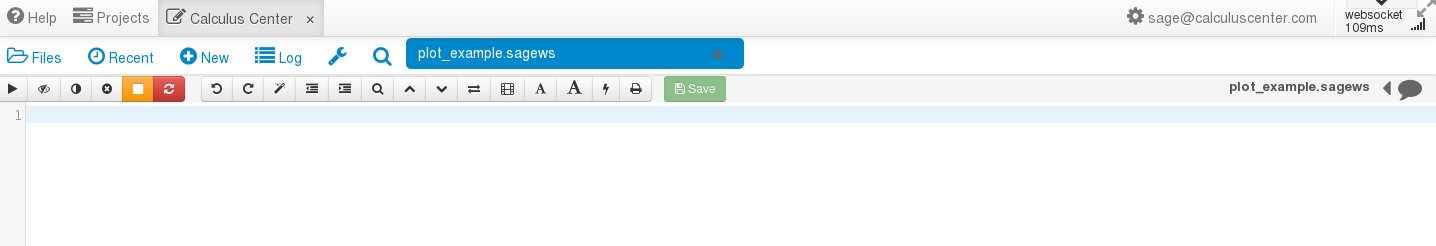• Now we can finally plot something.  Type f(x)=x^2 next to the "1" and then plot(f) right under it.  These are your plot commands.  To have Sage execute your commands you can hit shift+enter or click the right arrow button underneath the "Files" icon.  You should get this: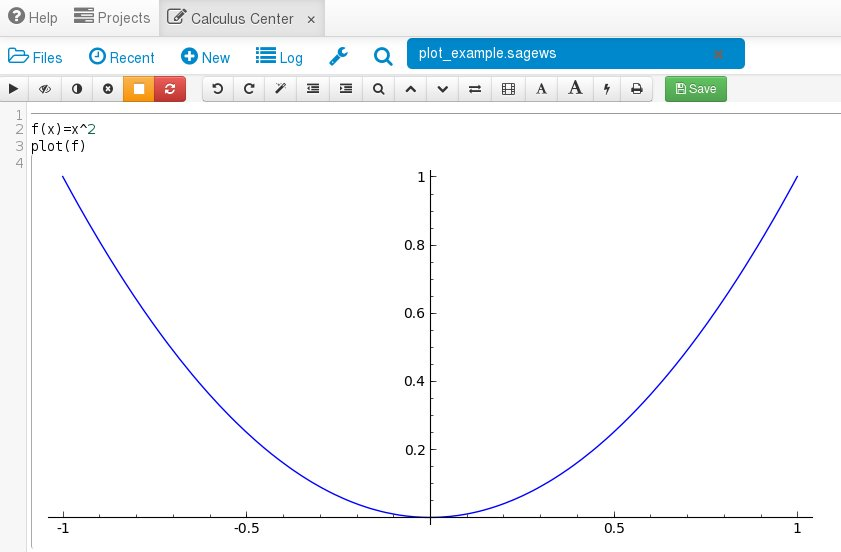Congratulations!  You have now made a plot in Sage.

One of the things to note is that the section with the commands to execute and the output is referred to as a cell.  The portion that appears to have a grey, left, open parenthesis is the output or result of running your commands.  A worksheet can have multiple cells.  Let's demonstrate that by typing more commands below the black horizontal line.  This will create a new cell in the worksheet.  Now here is a more complex plotting example: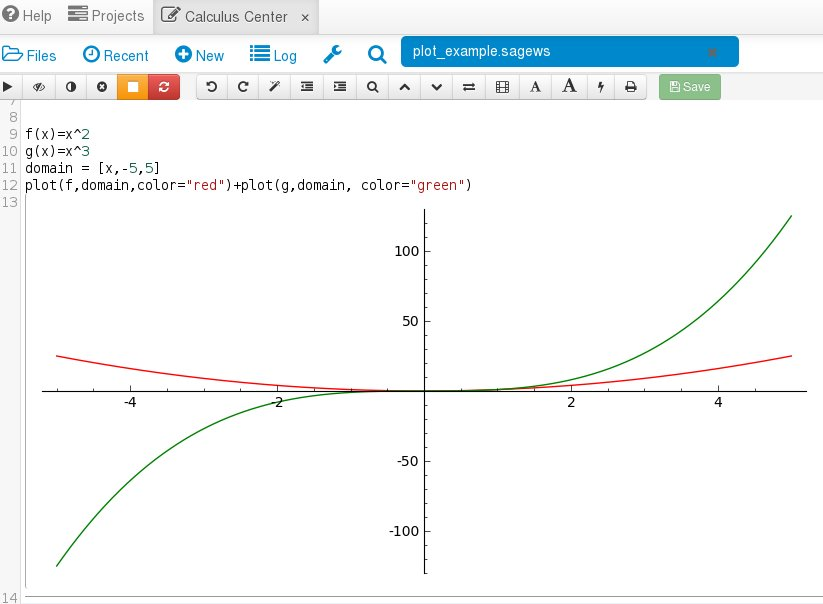In this example we did three additional things.  First, we added a second function on the plot.  Second, we changed where on the x-axis we make the plot with the domain variable.  Third, we changed the colors of the plots to distinguish the two functions from each other.

So let's recap what we learned:

• Projects are made up of worksheets and worksheets are made up of cells.
• Input commands like f(x)=x^2 and plot(f) into a worksheet cell and hit shift+enter to see the result next to the left, open parenthesis.
• More complex graphs can be made with commands like plot(f,domain,color="red)+plot(g,domain,color="green").

Tomorrow we will use Sage to differentiate a function. Finally, if you want to jump to the other blog posts for Sage Week then here they are: